# Beryl (Aqua Marine) cut normal to optic axis.

### 1. Beryl is uniaxial.

Figures 1 to 3 illustrates the conoscopy of a beryl crystal, uniaxial with negative optical sign. The figures are similar to the calcite images.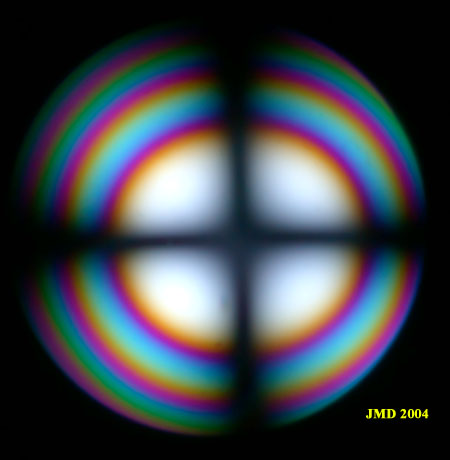Figure 1. Beryl section normal to optic axis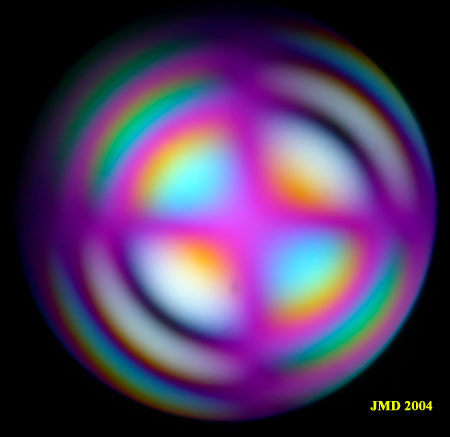Figure 2. Addition of wave plate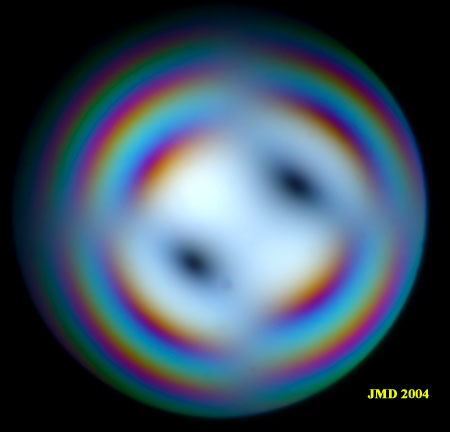Figure 3. Addition of quarter wave plate. Figures 2 and 3 indicate evidently a negative optic sign.

### 1. Beryl is sometimes pseudo biaxial.

Some beryl crystals exhibits conoscopic figures characteristic of biaxial minerals. Figures 4a and b below have been photographed at the position of maximum separation of the isogyres. The angle between the optic axes is estimated to be around 10°. The yellow and blue colors between the hyperbolae in  figures 5 b and c indicate clearly the negative optical sign in the usual way for biaxial minerals. When a quarter wave plate is added, figures 6 a and b are obtained. They seem to be similar to figure 3 for a uniaxial behavior except that the distance between the black dots is dependant on the rotation of the microscope stage. They can be interpreted on the basis of biaxial crystal optics.  In figure 6B, the dots appear like usual on the trace of the optic plane beyond the isogyres (compare figures 6B and 4B). To interpret figure 6a, remember that the retardation increases from the poles of the optic axes towards the center of the figure and then in a direction perpendicular to the optic plane. The melatopes in figure 4a are so close that the retardation equal to the quarter wave plate is not situated on  the optic plane but on the  line perpendicular. The retardations cancel on this line to produce the black spots observed. The same is true for the black arcs observed in the wave plate figure (5 a and b). The limit for the biaxial conoscopy for 2V angle going to zero is of course the uniaxial conoscopy. Thus the images below have characteristics common to both type of figures.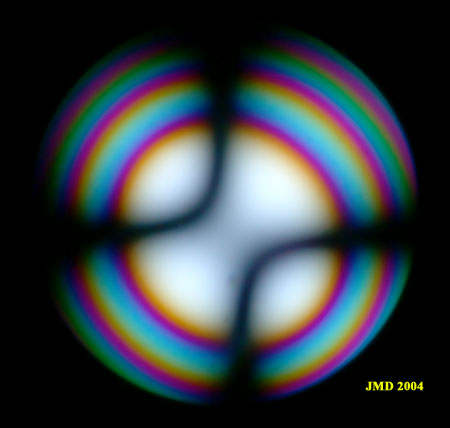Figure 4 a. Section of Beryl with biaxial behavior.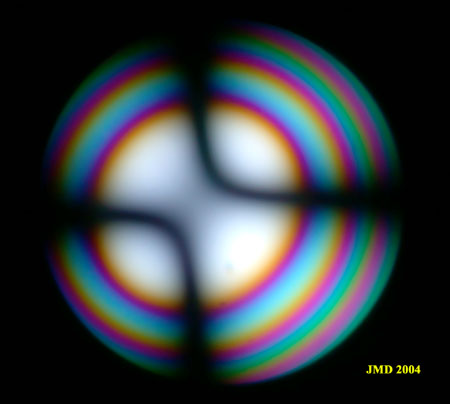Figure 4 B. Same section rotated by 90°.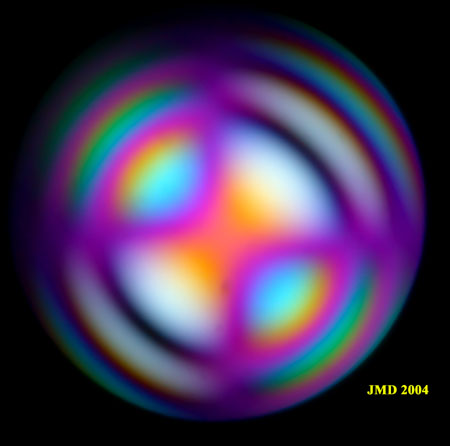Figure 5a. Addition of wave plate to figure 4a.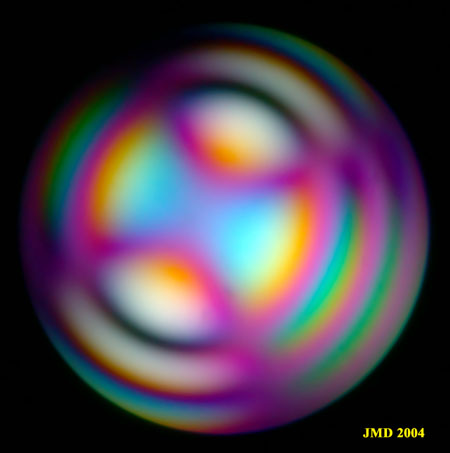Figure 5b. Addition of wave plate to figure 4b.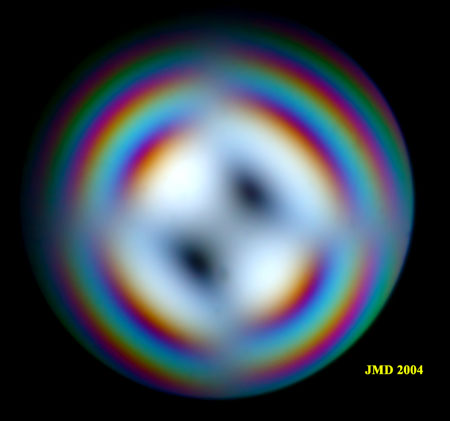Figure 6a. Addition of a quarter wave plate to figure 4a.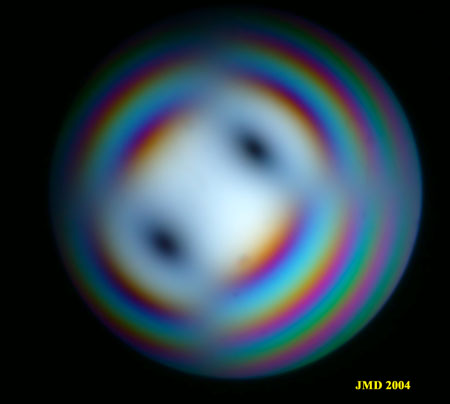Figure 6b. Addition of a quarter wave plate to figure 4b.